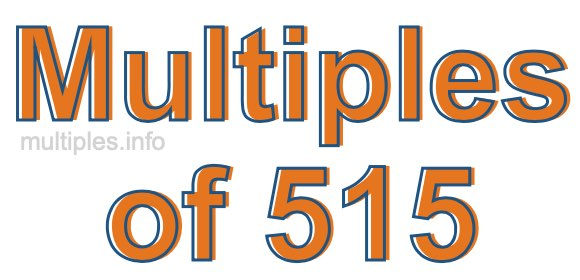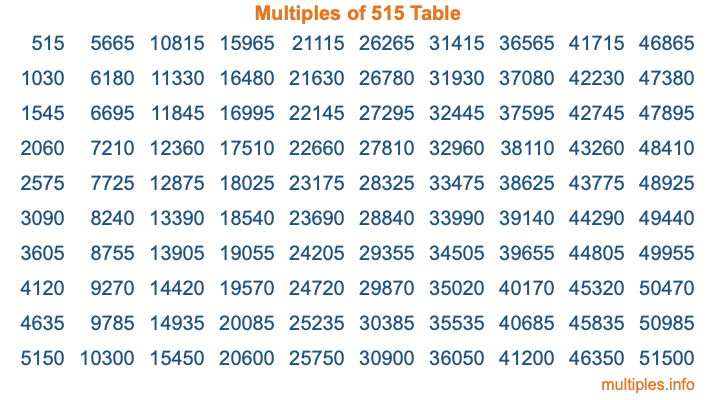Multiples of 515Welcome to the Multiples of 515 page. Here we will first teach you everything you will ever need to know about the multiples of 515, and then give you a study guide summary of everything we taught you to make sure you remember it all. Use this page to look up facts and learn information about the multiples of 515. This page will make you a multiples of five hundred fifteen expert!

Definition of Multiples of 515
Multiples of 515 are all the numbers that when divided by 515 equal an integer. Each of the multiples of 515 are called a multiple. A multiple of 515 is created by multiplying 515 by an integer.

Therefore, to create a list of multiples of 515, you start with 1 multiplied by 515, then 2 multiplied by 515, then 3 multiplied by 515, and so on for as long as you want. Thus, the list of the first five multiples of 515 is 515, 1030, 1545, 2060, and 2575. To see a larger list of multiples of 515, see the printable image of Multiples of 515 further down on this page. We also have a category where you can choose any nth multiple of 515.

Multiples of 515 Checker
The Multiples of 515 Checker below checks to see if any number of your choice is a multiple of 515. In other words, it checks to see if there is any number (integer) that when multiplied by 515 will equal your number. To do that, we divide your number by 515. If the the quotient is an integer, then your number is a multiple of 515.

Is  a multiple of 515?

Least Common Multiple of 515 and ...
A Least Common Multiple (LCM) is the lowest multiple that two or more numbers have in common. This is also called the smallest common multiple or lowest common multiple and is useful to know when you are adding our subtracting fractions. Enter one or more numbers below (515 is already entered) to find the LCM.

Check out our LCM Calculator if you need more details about the Least Common Multiple or if you need the LCM for different numbers for adding and subtraction fractions.

nth Multiple of 515
As we stated above, 515 is the first multiple of 515, 1030 is the second multiple of 515, 1545 is the third multiple of 515, and so on. Enter a number below to find the nth multiple of 515.

th multiple of 515

Multiples of 515 vs Factors of 515
515 is a multiple of 515 and a factor of 515, but that is where the similarities end. All postive multiples of 515 are 515 or greater than 515. All positive factors of 515 are 515 or less than 515.

Below is the beginning list of multiples of 515 and the factors of 515 so you can compare:

Multiples of 515: 515, 1030, 1545, 2060, 2575, etc.

Factors of 515: 1, 5, 103, 515

As you can see, the multiples of 515 are all the numbers that you can divide by 515 to get a whole number. The factors of 515, on the other hand, are all the whole numbers that you can multiply by another whole number to get 515.

It's also interesting to note that if a number (x) is a factor of 515, then 515 will also be a multiple of that number (x).

Multiples of 515 vs Divisors of 515
The divisors of 515 are all the integers that 515 can be divided by evenly. Below is a list of the divisors of 515.

Divisors of 515: 1, 5, 103, 515

The interesting thing to note here is that if you take any multiple of 515 and divide it by a divisor of 515, you will see that the quotient is an integer.

Multiples of 515 Table
Below is an image of the first 100 multiples of 515 in a table. The table is in chronological order, column by column. The first column has the first ten multiples of 515, the second column has the next ten multiples of 515, and so on.The Multiples of 515 Table is also referred to as the 515 Times Table or Times Table of 515. You are welcome to print out our table for your studies.

Negative Multiples of 515
Although not often discussed or needed in math, it is worth mentioning that you can make a list of negative multiples of 515 by multiplying 515 by -1, then by -2, then by -3, and so on, to get the following list of negative multiples of 515:

-515, -1030, -1545, -2060, -2575, etc.

Multiples of 515 Summary
Below is a summary of important Multiples of 515 facts that we have discussed on this page. To retain the knowledge on this page, we recommend that you read through the summary and explain to yourself or a study partner why they hold true.

There are an infinite number of multiples of 515.

A multiple of 515 divided by 515 will equal a whole number.

515 divided by a factor of 515 equals a divisor of 515.

The nth multiple of 515 is n times 515.

The largest factor of 515 is equal to the first positive multiple of 515.

515 is a multiple of every factor of 515.

515 is a multiple of 515.

A multiple of 515 divided by a divisor of 515 equals an integer.

515 divided by a divisor of 515 equals a factor of 515.

Any integer times 515 will equal a multiple of 515.

Multiples of a Number
Here you can get the multiples of another number, all with the same attention to detail as we did for multiples of 515 on this page.

Multiples of
Multiples of 516
Did you find our page about multiples of five hundred fifteen educational? Do you want more knowledge? Check out the multiples of the next number on our list!